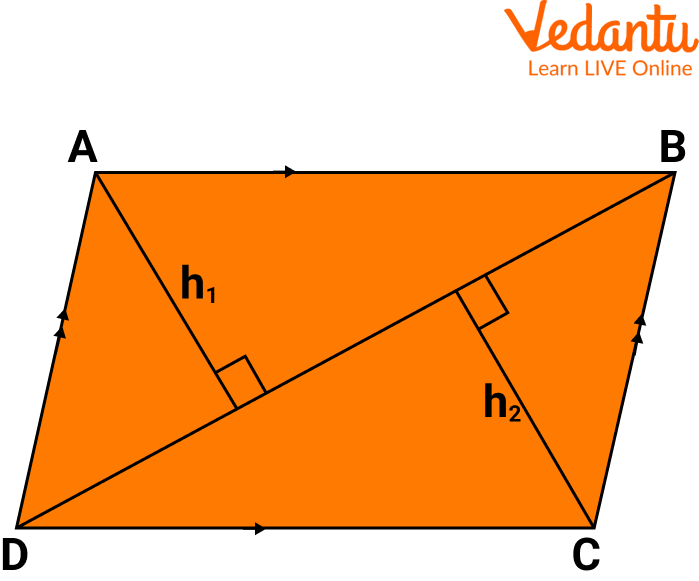Courses
Courses for Kids
Free study material
Offline Centres
More

# Area of Quadrilateral with Sides and DiagonalLast updated date: 01st Dec 2023
Total views: 98.1k
Views today: 1.98k## Introduction to the Area of Quadrilateral with Sides and Diagonal

In this article, we will learn about the general formula for the area of a quadrilateral with a diagonal calculator with the help of many solved examples. As we know that the area of a quadrilateral is the region enclosed by the sides of the quadrilateral. We are already acquainted with the term area.

It is defined as the region that is occupied inside the boundary of an object or figure. Its measurement is done in square units and the standard unit being square meters $\left(\mathrm{m}^2\right)$. Since we know that a polygon with four sides is called a quadrilateral. Hence, we shall learn about finding the area of a quadrilateral with sides and diagonals. A quadrilateral can be a square, rectangle, rhombus, parallelogram or trapezium.

Each of the quadrilaterals discussed above has its properties.

Though, some properties are common to all quadrilaterals.

They are as follows.

• They have four sides.

• They have four vertices.

• They have two diagonals.

• The sum of all interior angles is $360^{\circ}$.

## Area of Quadrilateral with Diagonal

The formula for the area of the quadrilateral can be found using different methods like dividing the quadrilateral into two triangles, using Heron’s formula or using the sides of the quadrilateral. So now let us discuss all methods in detail.

## Area of Quadrilateral by Dividing it into Two Triangles

• Consider a quadrilateral PQRS of different lengths; now, let us derive a formula for the area of a quadrilateral.

• We can see the quadrilateral as a combination of two triangles, with the diagonal PR being the common base.

• $h_{1}$ is the height of PSR and $h_{2}$ is the height of PQR, respectively.Area of the Quadrilateral with Diagonal

• The area of quad PQRS is equal to the sum of the area of triangle PSR and the area of triangle PQR.

• Area of triangle PSR $=\frac{(\text { base } \times h e I g h t)}{2}=\frac{\left(P R \times h_1\right)}{2}$ Area of triangle $P Q R=\frac{(\text { base } \times \text { height })}{2}=\frac{\left(P R \times h_2\right)}{2}$

• Thus, the area of quadrilateral $P Q R S$ is equal to the Area of triangle $\mathrm{PSR}+$ Area of triangle $\mathrm{PQR}=\frac{P R \times h_1}{2}+\frac{P R \times h_2}{2}=P R\left(\frac{h_1+h_2}{2}\right)$ $=\frac{1}{2} P R \times\left(h_1+h_2\right)$

• Hence the area of a general quadrilateral formula is, $=\frac{1}{2} \times$ diagonal $\times($ the sum of the height of two triangles $)$

Area of Quadrilateral with Diagonal Formula

Following are the area of quadrilateral with diagonal:

 Quadrilateral Area Formula Square $\mathrm{x}^2$ Rectangle $\mathrm{l} \times \mathrm{b}$ Parallelogram $\mathrm{b} \times \mathrm{h}$ Trapezoid $\frac{1}{2}(a+b) h$ Rhombus $\frac{1}{2} \times d_1 \times d_2$ Kite $\frac{1}{2} \times d_1 \times d_2$

## Solved Questions

Some solved examples based on the area of the quadrilateral with a diagonal calculator are given below:

Q 1. In the quadrilateral, ABCD is shown below, the side BD = 15 cm. The heights of the triangles ABD are 5cm and BCD 7 cm, respectively. Find its area.Ans:

Diagonal $=\mathrm{BD}=15 \mathrm{~cm}$

Given Heights, $h_1=5 \mathrm{~cm}$ and $h_2=7 \mathrm{~cm}$

Sum of the heights of the triangles $=h_1+h_2=5+7=12 \mathrm{~cm}$

Thus, the area of quadrilateral $A B C D$ is:

$A=12 \times$ diagonal $\times$ (Sum of the height of two triangles)

$=\frac{(15 \times 12)}{2}=90 \mathrm{~cm}^2$

Thus the area of the quadrilateral is $90 \mathrm{~cm}^2$.

Q 2. Find the sides and area of a square when the diagonal is given as $6 \mathrm{~cm}$.

Ans: Let us take a square of side $x$. If the square is divided into two right-angled triangles, then the hypotenuse of each triangle is equal to the diagonal of the square. As given, the diagonal is equal to $6 \mathrm{~cm}$.

According to Pythagoras's theorem,

$x^2+x^2=6^2$

$2 x^2=36$

$x^2=18$

$x=\sqrt{18}$

$x=3 \sqrt{2}$ units

To find the area of a square when a diagonal is given, we can use any of the below methods:

$\text { Area of a square }=\text { side } \times \text { side }=3 \sqrt{2} \times 3 \sqrt{2}$

$=9 \times 2=18 \mathrm{~cm}^2$

## Practice Questions

Q1. Calculate the area of a quadrilateral with the given measurements:

Perpendicular heights = 60 m and 20 m, Diagonal = 50 m

Solution: Area $=2000 \mathrm{~m}^2$

Q2. A quadrilateral has four equal sides each of length $4 \mathrm{~cm}$. Find its area?

Solution. Area $=16 \mathrm{~m}^2$

Q3. The length of a quadrilateral (rectangle) is $6 \mathrm{~m}$ and the breadth is $5 \mathrm{~m}$. Find the area of the rectangle.

Solution: Area $=30 \mathrm{~m}^2$

## Summary

In this article, we have discussed the area of quadrilaterals with a diagonal formula for different types of quadrilaterals. These formulas help you to calculate the area of a rectangle, square, parallelogram, rhombus, and trapezium. We have also discussed the area of the quadrilateral with a diagonal calculator. A quadrilateral can also be divided into two triangles, calculating the area of each triangle given its vertices, and then adding these values to get the required total area of the quadrilateral with sides and diagonals.

## FAQs on Area of Quadrilateral with Sides and Diagonal

1. Name the unit of the area of a quadrilateral.

The Unit of the area of a quadrilateral is the same as other shapes, that is, square units of length (square meter, square inches, etc.)

2. How do you calculate the area of an irregular shape?

To find the area of an irregular shape, we first break the shape into common shapes. Then we find the area of each shape and add them. For example, if an irregular polygon is made up of a square and a triangle, then: Area of an irregular polygon = Area of Square + Area of Triangle.

3. What is a shape with 7 sides called?

A shape with 7 sides is called a heptagon.